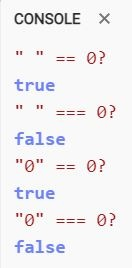# Difference between double equals and triple equals

What is the difference between == and ===? How double equals differs from triple equals?## Double equals and triple equals

They are both checking the equality. Double equals does not care about data type. Triple equals does care about data types.

We can say that double equls checks equality but triple equals check strong equality.

`"0" == 0`
is true
`"0" === 0`
is false because string does not equals to number
Also one more weird thing. Javascript thinks empty string equals zero. When checking strong equality with triple equals it is false.
`" " == 0`
is true because Javascript consides empty string as zero
`" " === 0`
is false
Please remember about that writing code. Use triple equals where possible to avoid bugs.

## When to use triple equals

• You should use triple equals (`===`) when you need to check for strong equality. This is especially important when you are working with different data types.
• For example, you would use triple equals to check if two strings are equal, even if they are empty strings.